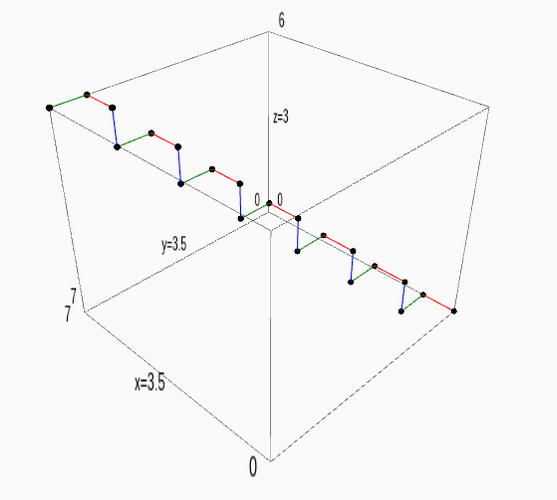# Plotting 3D points in a certain way

I wish to recreate this sort of plot: http://i.imgur.com/19UPf5D.png i.e, plot 3d points in the xyz plane and connect the points by a line.

Does anyone know how to do that?

I found: http://doc.sagemath.org/html/en/refer... but I haven't been able to find exactly what I want.

edit retag close merge delete

Sort by » oldest newest most votedMaybe you can try something of the form

# list of points
pts = [(0, 0, 0), (1, 0, 0), (1, 1, 0), (1, 1, 1), (2, 1, 1), (2, 2, 1), (2, 2, 2), (3, 2, 2), (3, 3, 2), (3, 3, 3), (4, 3, 3), (4, 4, 3), (4, 4, 4), (5, 4, 4), (5, 5, 4), (5, 5, 5), (6, 5, 5), (6, 6, 5), (6, 6, 6), (7, 6, 6), (7, 7, 6)]

# some red segments
red_segments = [[pts[i],pts[i+1]] for i in range(len(pts)-1) if i%3==0]
# some green segments
green_segments = [[pts[i],pts[i+1]] for i in range(len(pts)-1) if i%3==1]
# some blue segments
blue_segments = [[pts[i],pts[i+1]] for i in range(len(pts)-1) if i%3==2]

# empty graphics object
P=Graphics()

for rs in red_segments:
P += line3d(rs,color='red')
for gs in green_segments:
P += line3d(gs,color='green')
for bs in blue_segments:
P += line3d(bs,color='blue')

P+=point3d(pts,color='black')
P


Sample output:Update: Removed bug on 2nd line of code and added a last line (curiously P.show()does not actually show the plot in cloud.sagemath.com).

more

Hi fidbc, I attempted to run your code, but encountered errors. In what environment did you run this in? Error (This is Ozera, but the Ozera account wouldn't let me post a comment)

Sorry @Ozera, @Nomly. My code had a bug but I think it works now. I'm running this code in cloud.sagemath.com

@fidbc How odd. Do you know why replacing it with simply 'P' is the solution. In the documentation I read, it was always using the show() function. Also, is there a way to remove the bounding box? I'm not sure where the frame=False argument would go.

@Nomly It seems that show(P,frame=False) works fine. Maybe this is a bug, I'll ask in the sage-support group.